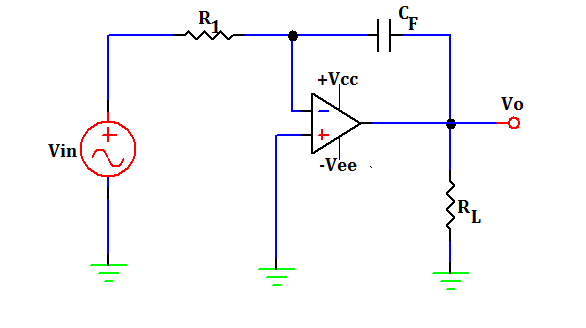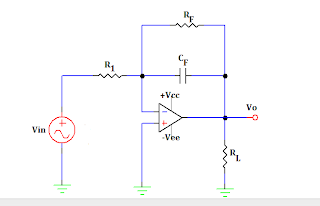# OP AMP INTEGRATOR CIRCUIT DESIGN AND WORKING

The OP AMP Integrator, is a circuit which performs the integration of an input signal. The output of an integrator will be the mathematical integral value of continuous input signal. Such a circuit is obtained by using a basic inverting amplifier configuration if the feedback resistor Rf is replaced by a capacitor Cf.

Vo = -1/R1CF  ∫0T(Vin dt + C)

The above equation shows that the output voltage is directly proportional to the negative integral of input voltage and inversely proportional to the time constant R1Cf.

For Example:-

• If the input is a sinewave, the output will be a cosine wave.

• If the  input  to the integrator is square wave, the output will be a triangular wave.When Vin=0, the integrator shown in figure will act as an open loop amplifier. This is because the capacitor Cf acts as an open circuit (The impedance of feedback capacitor approximates to infinity) to the input offset voltage Vio. In other words, the input offset voltage Vio and the part of the input current charging capacitor Cf produces the error voltage at the output of the integrator.In PRACTICAL INTEGRATOR, to reduce the error voltage at the output, a resistor Rf is connected across the feedback capacitor Cf. Thus, Rf limits the low frequency gain and hence minimizes the variations in the output voltage. Both the stability and Roll-off problems can be eliminated by the addition of a resistor Rf in the practical Integrator.

Note:-

• STABILTY: The term stability refers to the constant gain as frequency of an input signal is varied over a certain range.

• Roll-off: The term Roll-off refers to the rate of decrease in gain at lower frequencies.

INTEGRATOR DERIVATION

By applying Kirchoff’s current equation at node V2,

I1 = IB + IF

The input bias current IB is negligibly small so we can ignore it,
I1 ≈ IF

Current through the feedback capacitor,

IC = C dVc /dt

Therefore,

Vin-V2/R1 = CF (d/dt) (V2-V0)

However V1 = V2 = 0 because A (Gain) is very large.

Therefore,

Vin/R1 = CF d/dt (-V0)

=CF (-V0) + V0   at t=0

Vo = -1/R1CF  ∫0T(Vin dt + C)

Where C is the integration constant.### Tittu

#### Author & Editor

I'm Tittu Thomas, An Electronics and Communication Engineer from India, Kerala. I love doing hobby electronics circuits, blogging, programming, etc. Started this blog while doing my B-Tech degree under CUSAT university.